# Place Value (Tens and Ones)

Times up!
Times up! You may finish answering the current question and then proceed to your score!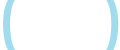Sorry, you have used all of your available hints for this lesson.
Practice Limit Reached

You've reached your daily practice limit of 12 questions.

Award InformationAwarded toQuestion Hint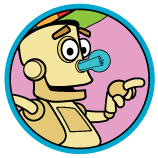Choose Family Member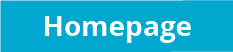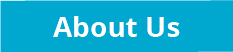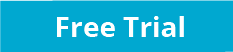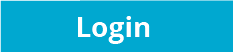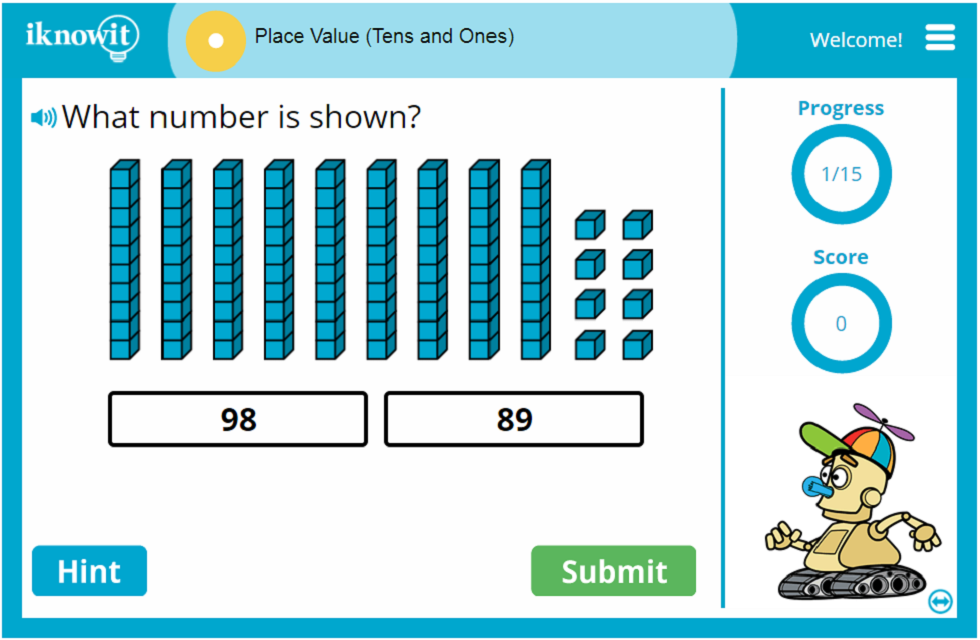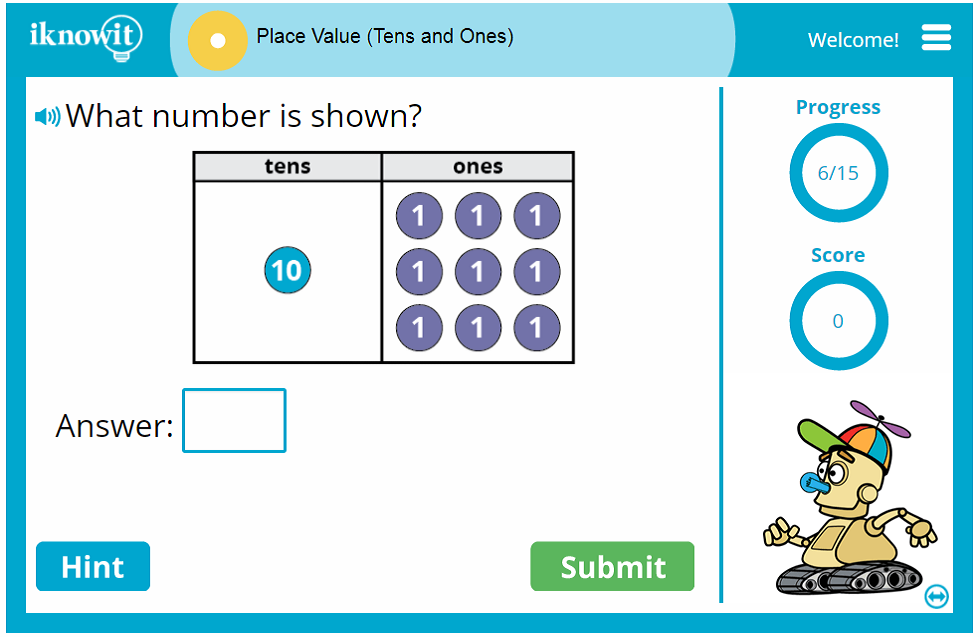## Place Value Math Lesson

Welcome to iKnowIt.com, a site where elementary students can practice their math skills. In the place value lesson above, students will demonstrate an ability to:

* Use images of place value blocks to find the number of tens and ones in a given number.

* Match written words to numbers (example: twelve = 12)

* Identify the number of tens and ones in a two-digit number (example: 79 = 7 tens and 9 ones)

* Add multiples of ten to a single-digit number to make a two-digit number (example: 60 + 9 = 69)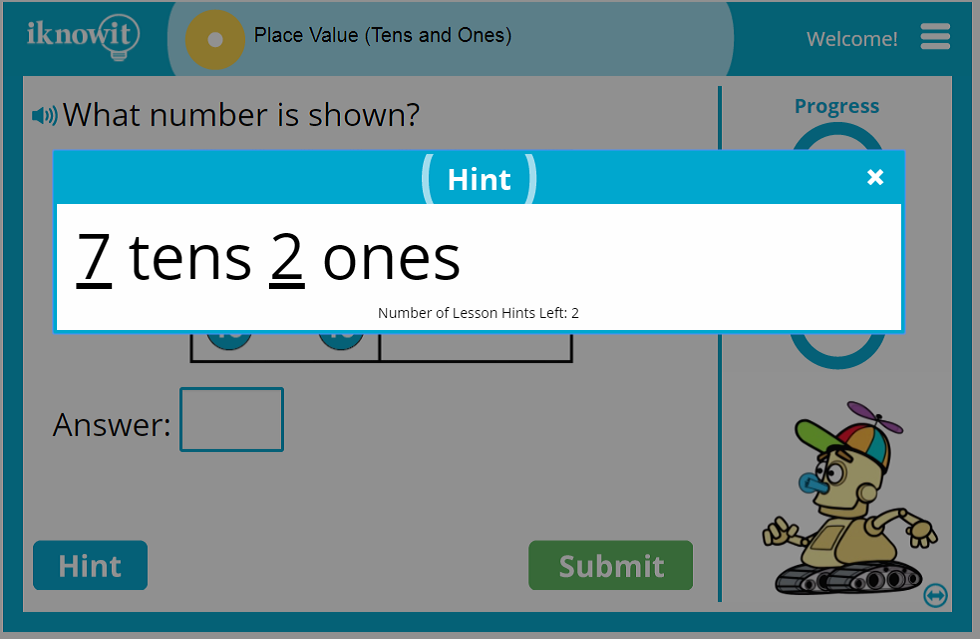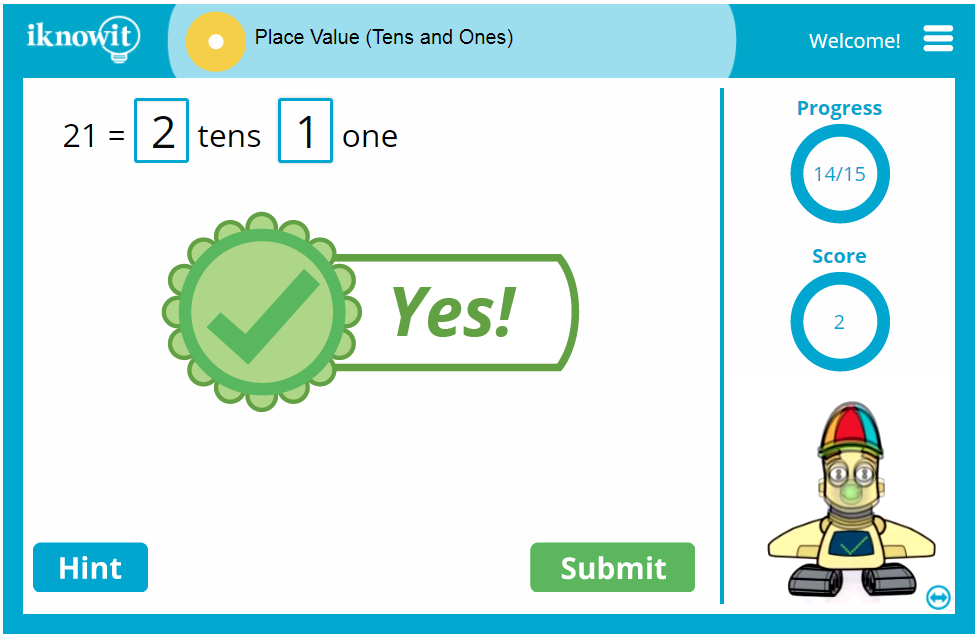This interactive math activity has a variety of question types, including fill-in-the-number questions, and multiple choice questions.

If a student answers correctly, the animated robot character will do an adorable or funny dance. He actually knows over 20 dance moves. The more students answer correctly, the more they will see. The circles on the right-hand part of the screen are used to track the score.

When students have difficulty figuring out the answer, they can click on the "hint" button. Remind your class to use hints sparingly because they're only allowed three during the course of the game.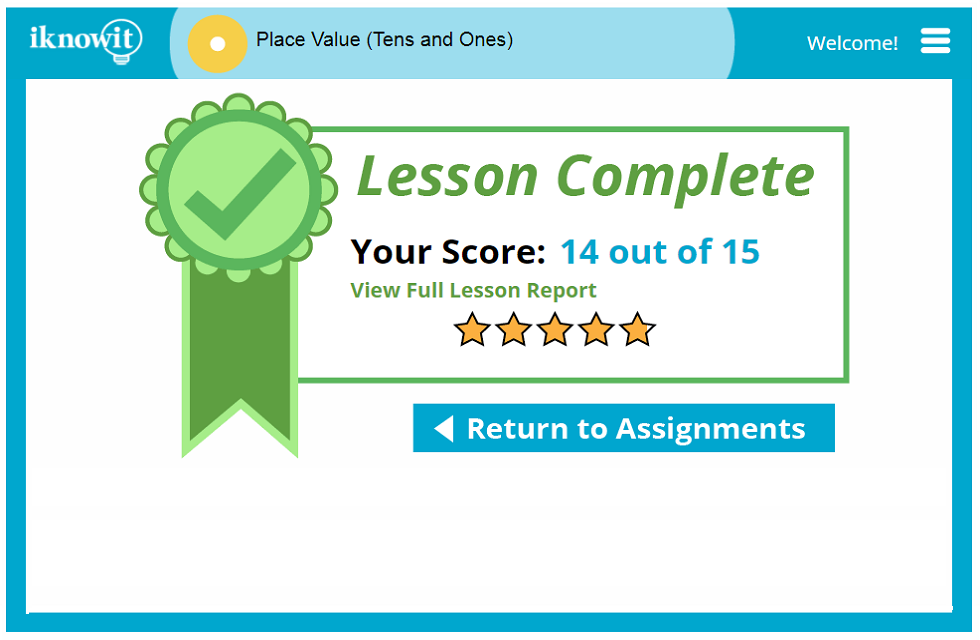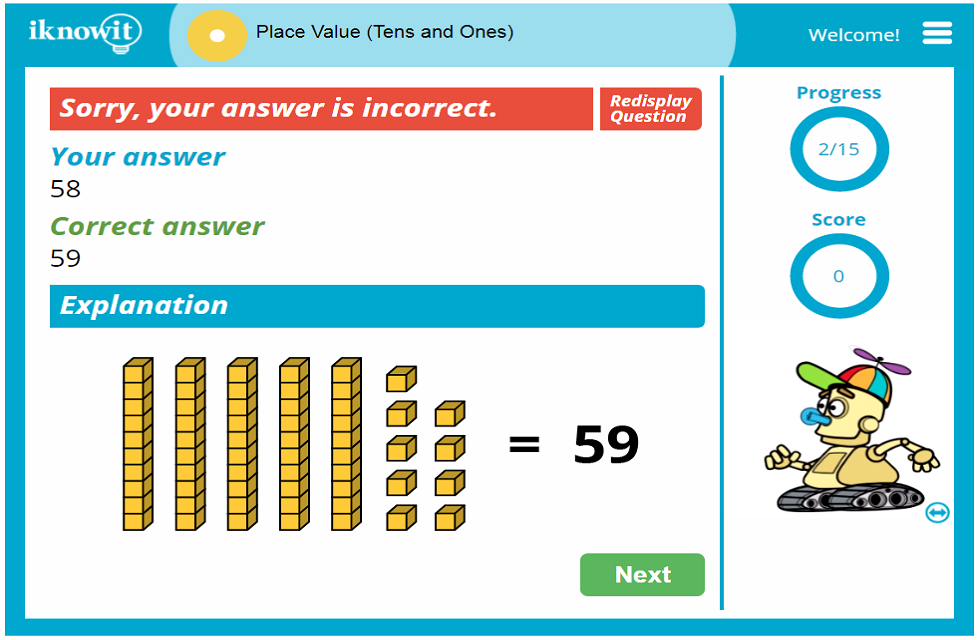## Level

We've classified this as a Level A lesson that may be appropriate for 1st or 2nd grades.

## Common Core Alignment

1.NBT.2
Operations & Numbers (Base Ten)

Know that the both digits of a two-digit number show tens and ones. 10 can be represented as a stack of ten ones - called a "ten stick." Numbers 11 through 19 are composed of a "ten stick" and one, to nine individual ones. Two-digit numbers ending in zero (10, 20, 30, 40, 50, 60, 70, 80, 90) actually represent one, two, three, four, five, six, seven, eight, or nine tens (with 0 ones).

## You might also be interested in...

Ordering Numbers Up to 20 (Level A)
Drag numbers around the screen to order them from least to greatest and vice-versa.

Fractions of Shapes (Level A)
Tell what fraction of each shape is colored in. This graphical activity makes learning basic fractions fun and easy.